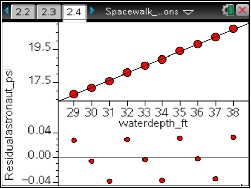# Activities

••• ##### Subject Area

• Math: Statistics: Describing Bivariate Data

• ##### Author9-12

50 Minutes

• ##### Device
•TI-Nspire™ CX/CX II
•TI-Nspire™ CX CAS/CX II CAS
• TI-Nspire™
• TI-Nspire™ CAS

2.1

## NASA - Spacewalk Training#### Activity Overview

In this activity, students will plot data, looks at patterns, and draw conclusions given a real-world context of astronauts training in the Neutral Buoyancy Laboratory (NBL) in Houston, TX.

#### Objectives

Students will:

• explain what is meant by the direction, form and strength of the overall pattern of a scatter plot
• define the correlation coefficient, r, and describe what it measures
• write a regression equation and interpret the meaning of the slope and y-intercept in context of the problem
• explain what is meant by extrapolation and interpret a situation where extrapolation occurs
• make predictions based on the correct mathematical model
• plot residuals and interpret their graphical form.